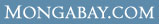# WHAT ARE THE AVERAGE Wages & salaries for Computer and mathematical occupations in Tucson, AZ ?

 Overview Every year U.S. Department of Labor's Bureau of Labor Statistics releases employment and wage figures. This data was released in May 2006 and covers Wages & salaries for Computer and mathematical occupations in Tucson, AZ Computer and mathematical occupations (Occupation [OCC] code: 15-0000) in Tucson, AZ (BLS Area code: 46060 ) earned an average wage of \$29.56 per hour and an average annual salary of \$61,490 The BLS estimates that in Tucson, AZ there were around 7,630 employed in the field of Computer and mathematical occupations.

Industry Comparison
Industry comparison
Computer and mathematical occupations in Tucson, AZ earned 11% less than average for Computer and mathematical occupations in the United States.

Region/area/city comparison
Computer and mathematical occupations in Tucson, AZ earned 69% more than the average worker in Tucson, AZ.

State salary comparison
Computer and mathematical occupations in Tucson, AZ earned 40% more than the average worker in Arizona.

National salary comparison
Computer and mathematical occupations in Tucson, AZ earned 57% more than the average worker in the United States.

Wage and salary details
More detailed employment information for Computer and mathematical occupations in Tucson, AZ

Hourly wage data for Computer and mathematical occupations in Tucson, AZ

 Mean hourly wage: \$29.56 Tenth (10th) percentile hourly wage: \$15.66 Twenty-fifth (25th) percentile hourly wage: \$20.37 Fiftieth (50th) percentile wage [median] hourly wage: \$28.46 Seventy-fifth (75th) percentile hourly wage: \$37.06 Ninetieth (90th) percentile hourly wage: \$45.93

Salary figures for Computer and mathematical occupations in Tucson, AZ

 Mean annual salary: \$61,490 Tenth (10th) percentile annual salary: \$32,560 Twenty-fifth (25th) percentile annual salary: \$42,370 Fiftieth (50th) percentile wage [median] annual salary: \$59,200 Seventy-fifth (75th) percentile annual salary: \$77,090 Ninetieth (90th) percentile annual salary: \$95,530

Indexwhat's new | rainforests home | help support the site | madagascar | search | about | contact